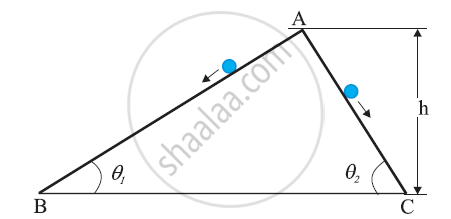# ISC (Arts) Class 11 - CISCE Question Bank Solutions for Physics (Theory)

Subjects
Topics
Subjects
Popular subjects
Topics
Physics (Theory)
< prev  1 to 7 of 7  next >

Consider the situation of the previous problem. Take "the table plus the ball" as the system. friction between the table and the ball is then an internal force. As the ball slows down, the momentum of the system decreases. Which external force is responsible for this change in the momentum?

 Motion of System of Particles and Rigid Body
Chapter:  Motion of System of Particles and Rigid Body
Concept: Momentum Conservation and Centre of Mass Motion

Write the SI units of speed and average speed.

 Kinematics
Chapter:  Kinematics
Concept: Instantaneous Velocity and Speed

Two inclined frictionless tracks, one gradual and the other steep meet at A from where two stones are allowed to slide down from rest, one on each track . Will the stones reach the bottom at the same time? Will they reach there with the same speed? Explain. Given θ1 = 30°, θ2 = 60°, and = 10 m, what are the speeds and times taken by the two stones?Work, Power and Energy
Chapter:  Work, Power and Energy
Concept: Conservation of Mechanical Energy

If the density of a solution is 3.12 g mL–1, the mass of 1.5 mL solution in significant figures is ______.

 Physical World and Measurement
Chapter:  Physical World and Measurement
Concept: Significant Figures

Explain what is Doppler effect in sound

 Oscillations and Waves
Chapter:  Oscillations and Waves
Concept: Wave Motion

Show that the child’s new kinetic energy of rotation is more than the initial kinetic energy of rotation. How do you account for this increase in kinetic energy?

 Motion of System of Particles and Rigid Body
Chapter:  Motion of System of Particles and Rigid Body
Concept: Moment of Inertia

Two sound waves travel at a speed of 330 m/s. If their frequencies are also identical and are equal to 540 Hz, what will be the phase difference between the waves at points 3.5 m from one source and 3 m from the other if the sources are in phase?

 Oscillations and Waves
Chapter:  Oscillations and Waves
Concept: Beats
< prev  1 to 7 of 7  next >